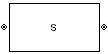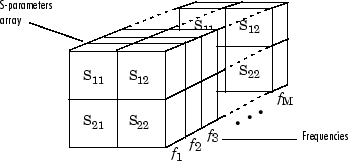# S-Parameters Passive Network

Model passive network using S-parameters

• Library:
• RF Blockset / Equivalent Baseband / Black Box Elements

•## Description

The S-Parameters Passive Network block models the two-port passive network described in the block dialog box, in terms of its S-parameters and the frequencies and reference impedance of the S-parameters.

In the S-Parameters field of the block dialog box, provide the S-parameters for each of the M frequencies as a 2-by-2-by-M array. In the Frequency field, specify the frequencies for the S-parameters as an M-element vector. The elements of the vector must be in the same order as the S-parameters. The figure shows the correspondence between the S-parameters array and the vector of frequencies.The S-Parameters Passive Network block interpolates the given S-parameters to determine their values at the modeling frequencies. The Output Port block determines the modeling frequencies. See Map Network Parameters to Modeling Frequencies for more details.

## Parameters

expand all

### Main

S-parameters for a two-port passive network, specified as a 2-by-2-by-M array where M is the number of S-parameters.

Frequency of S-parameters, specified as an M-element vector with each element unit in hertz. All frequencies must be positive.

Reference impedance of network, specified as a nonnegative scalar in ohms or vector of length M with each element unit in ohms. The value of this parameter can be real or complex. If you provide a scalar value, then that value is applied to all frequencies.

Method to interpolate the network parameters, specified as one of the following:

MethodDescription
`Linear`Linear interpolation
`Spline`Cubic spline interpolation
`Cubic`Piecewise cubic Hermite interpolation

### Visualization

Frequency data source, specified as ```Same as the S-Parameters``` or `User-specified`.

Frequency data range, specified as a vector with each element unit in hertz.

Reference impedance, specified as a nonnegative scalar in ohms.

Type of data plot to visualize using the given data, specified as one of the following:

• `X-Y plane` — Generate a Cartesian plot of the data versus frequency. To create linear, semilog, or log-log plots, set the Y-axis scale and X-axis scale accordingly.

• `Composite data` — Plot the composite data.

• `Polar plane` — Generate a polar plot of the data. The block plots only the range of data corresponding to the specified frequencies.

• `Z smith chart`, ```Y smith chart```, and `ZY smith chart` — Generate a Smith® chart. The block plots only the range of data corresponding to the specified frequencies.

Type of S-Parameters to plot, specified as one of the following. When noise is spectral, `NF` plotting is possible.

 `S11` `S12` `S21` `S22` `GroupDelay` `GammaIn` `GammaOut` `VSWRIn` `VSWROut` `OIP3` `IIP3` `NF` `NFactor` `NTemp` `TF1` `TF2` `TF3` `Gt` `Ga` `Gp` `Gmag` `Gmsg` `GammaMS` `GammaML` `K` `Delta` `Mu` `MuPrime`

Type of S-Parameters to plot, specified as one of the following. When noise is spectral, `NF` plotting is possible.

 `S11` `S12` `S21` `S22` `GroupDelay` `GammaIn` `GammaOut` `VSWRIn` `VSWROut` `OIP3` `IIP3` `NF` `NFactor` `NTemp` `TF1` `TF2` `TF3` `Gt` `Ga` `Gp` `Gmag` `Gmsg` `GammaMS` `GammaML` `K` `Delta` `Mu` `MuPrime`

Plot format, specified as one of the following.

 `dB` `Magnitude (decibels)` `Abs` `Mag` `Magnitude (linear)` `Angle` `Angle(degrees)` `Angle(radians)` `Real` `Imag` `Imaginary`

Plot format, specified as one of the following.

 `dB` `Magnitude (decibels)` `Abs` `Mag` `Magnitude (linear)` `Angle` `Angle(degrees)` `Angle(radians)` `Real` `Imag` `Imaginary`

Frequency plot, specified as `Freq`.

Frequency plot format, specified as one of the following.

 `Auto` `Hz` `kHz` `MHz` `GHz` `THz`

Y-axis scale, specified as `Linear` or `Log`.

X-axis scale, specified as `Linear` or `Log`.

Plot specified data using the plot button.